# Test: Utilization of Electrical Energy- 2

## 20 Questions MCQ Test Mock Test Series for SSC JE Electrical Engineering | Test: Utilization of Electrical Energy- 2

Description
Attempt Test: Utilization of Electrical Energy- 2 | 20 questions in 12 minutes | Mock test for SSC preparation | Free important questions MCQ to study Mock Test Series for SSC JE Electrical Engineering for SSC Exam | Download free PDF with solutions
QUESTION: 1

### In dielectric heating, the current flows through:

Solution:

Upon application of an alternating electrical field, a displacement current caused by polarization and a conduction current caused by the presence of free, electrically charged particles appear in the dielectric. The flow of the total current causes the liberation of heat.

QUESTION: 2

### In induction heating, the depth up to which the current will penetrate is proportional to:

Solution:

The depth of penetration of induced currents into the disc is given by: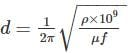Where, ρ is the specific resistance in Ω-cm

f is the frequency in Hz

μ is the permeability of the charge

The depth up to which the current will penetrate is inversely proportional to square of the frequency

QUESTION: 3

### In submerged arc furnaces, the power is controlled by:

Solution:

In submerged arc furnaces, the power is controlled by

1. By varying the length of the arc by raising or lowering the electrodes thereby varying the resistance of the arc

2. By varying the applied voltage by the use of tappings provided on the primary side of furnace transformer

QUESTION: 4

In direct arc furnace, which of the following is of high value?

Solution:

In direct arc furnace, when supply is given to the electrodes, two arcs are established and current passes through the charge. The arc is in direct contact with the charge and heat is also produced by current flowing through the charge itself. In direct arc furnace current value is high.

QUESTION: 5

During the resistance welding, the heat produced at the joint is proportional to:

Solution:

Resistance welding is the process of joining two metals together by the heat produced due to the resistance offered to the flow of electric current at the junctions of two metals. The heat produced by the resistance to the flow of current is given by

H = I2R

The heat produced at the joint is proportional to I2R

QUESTION: 6

The main drawbacks of the resistance welding are:

Solution:

The main drawbacks of the resistance welding are:

1. Initial cost is very high
2. High maintenance cost
3. The workpiece with heavier thickness cannot be welded, since it requires high input current
QUESTION: 7

In the spot welding:

Solution:

Spot welding means the joining of two metal sheets and fusing them together between copper electrode tips at suitably spaced intervals by means of heavy electric current passed through the electrodes.

It is desirable to clean the sheets thoroughly before welding.

The welding current varies widely depending upon the thickness of and composition of plates. It varies from 1000 to 10000 A and voltage between the electrodes is usually less than 2 V.

QUESTION: 8

The length of arc required depends on:

Solution:

The length of the arc required for welding depends upon the following factors:

1. The surface coating and the type of electrodes used

2. The position of welding

3. The amount of current used

QUESTION: 9

A substance that changes its electrical resistance when illuminated by light is called:

Solution:

Photoconductivity is an optical and electrical phenomenon in which a material becomes more electrically conductive due to the absorption of electromagnetic radiation such as visible light, ultraviolet light, infrared light, or gamma radiation

Photovoltaic is a method for generating electric power by using solar cells to convert energy from the sun into a flow of electrons by the photovoltaic effect

Photoelectric effect is the emission of electrons or other free carriers when light shines on a material

QUESTION: 10

The illumination of various points on a horizontal surface illuminated by the same source varies as:

Solution:

The illumination of the surface is,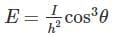Where, h is the height of source from the surface in m

I is the luminous intensity on candela

The above equation is also known as cosine cube law. This law states that the illumination at any point on a surface is dependent on the cube of cosine of the angle between lines of flux and normal of that point.

QUESTION: 11

In the electric discharge lamps, the light is produced by:

Solution:

The lamps that emit light of the colour produced by discharge takes place through the gas or vapour present in the discharge tube such as neon gas, sodium vapour, mercury vapour, etc.

QUESTION: 12

An auto-transformer used with a sodium vapour lamp should have:

Solution:

To start sodium vapour lamp, 380 - 450 V of striking voltage required for 40 - 100 W lamps. These voltages can be obtained from a high reactance transformer or an auto transformer.

QUESTION: 13

Which law states that the mass of substance liberated from an electrolyte is proportional to the quantity of electricity passing through it?

Solution:

This law states that the mass of substance deposited from an electrolyte is proportional to the quantity of electricity passing through the electrolyte in the given time

m ∝ It

This law states that when the same quantity of electric current is passed through different electrolytes, the masses of the substances deposited are proportional to their respective chemical equivalents or equivalent weights.

QUESTION: 14

The process of depositing one metal over the other metal is known as:

Solution:

Electrodeposition: It is the process by which one metal is deposited over other metal or non-metal by electrolysis.

Electro metallization: It is the process by which the metal can be deposited on a conduction base for decoration and for protective purposes. Any non-conductive base is made as conductive by depositing graphite layer over it.

Anodizing: The process of deposition of oxide film on a metal surface is known as anodizing and oxidation.

Electrofacing: It is the process of the coating of a metallic surface with a harder metal by electrodeposition

QUESTION: 15

The speed-time curve for the urban service has no:

Solution:

In urban service, there is no free running period. The distance between two stops is less about 1 km. Hence relatively short coasting and longer braking period is required.

QUESTION: 16

The speed of a train estimated taking into account the stoppage time at a station in addition to the actual running time between stops is called the:

Solution:

Average Speed: It is the mean of the speeds attained by the train from start to stop, i.e. it is defined as the ratio of the distance covered by the train between two stops to the total time of run. It is denoted with Va

Average speed = distance between stops/actual time of run

Va = D/T

Where Vis the average speed of train in kmph

D is the distance between stops in km

T is the actual time in hours

Schedule Speed: The ratio of the distance covered between two stops to the total time of the run including the time for stop is known as schedule speed. It is denoted with Vs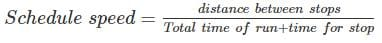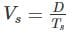Where Ts is the schedule time in hours

QUESTION: 17

The coefficient of the adhesion is the ratio of tractive effort to slip the wheels and:

Solution:

Coefficient of adhesion (μ): It is defined as the ratio of the tractive effort (Ft) to propel the wheel of a locomotive to its adhesive weight (W).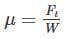QUESTION: 18

Load equalization is desirable in the case of

Solution:

The load fluctuations take place in many of the industrial drives such as rolling mills, electric hammers, presses, and reciprocating pumps, where the load on the motor varies widely within a span of few seconds. The sudden and peak load requires very large current from the supply results high voltage drop in the system or alternately would require very large size of cables. It is very essential to smooth out fluctuating load is known as load equalization.

QUESTION: 19

Which of the following material is most commonly used for the filaments in incandescent lamps?

Solution:

Tungsten is most commonly used for the filaments in incandescent lamps.

QUESTION: 20

Centrifugal pumps are usually driven by:

Solution:

Centrifugal pumps are used to transport fluids by the conversion of rotational kinetic energy to the hydrodynamic energy of the fluid flow. The rotational energy typically comes from an engine or electric motor. Squirrel cage induction motors are used for this purpose.Use Code STAYHOME200 and get INR 200 additional OFF Use Coupon Code